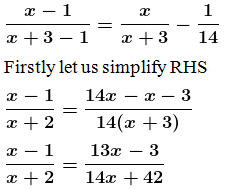Guru

# In a certain positive fraction, the denominator is greater than the numerator by 3. If 1 is subtracted from both the numerator and denominator, the fraction is decreased by 1/14. Find the fraction.

• 0

This question has been taken from Book:- ML aggarwal, Avichal publication, class10th, quadratic equation in one variable, chapter 5, exercise 5.5
This is an important ques.

In a certain positive fraction, the denominator is greater than the numerator by 3.

If 1 is subtracted from both the numerator and denominator,

the fraction is decreased by 1/14. Find the fraction.

Question no.9 , ML Aggarwal, chapter 5, exercise 5.5, quadratic equation in one variable, ICSE board,

Share

1. Solution:

Let the numerator be ‘x’

Denominator be ‘x+3’

So the fraction is x/(x+3)

According to the question,By cross multiplying, we get

(x – 1) (14x + 42) = (x + 2) (13x – 3)

14x2 + 42x – 14x – 42 = 13x2 – 3x + 26x – 6

14x2 + 42x – 14x – 42 – 13x2 + 3x – 26x + 6 = 0

x2 + 5x – 36 = 0

let us factorize,

x2 + 9x – 4x – 36 = 0

x(x + 9) – 4 (x + 9) = 0

(x + 9) (x – 4) = 0

So,

(x + 9) = 0 or (x – 4) = 0

x = -9 or x = 4

So the value of x = 4 [since, -9 is a negative number]

When substitute the value of x = 4 in the fraction x/(x+3), we get

4/(4+3) = 4/7

∴ The required fraction is = 4/7

• 0